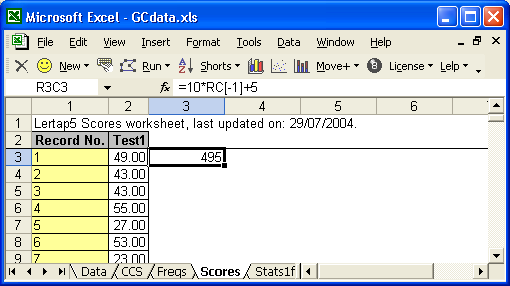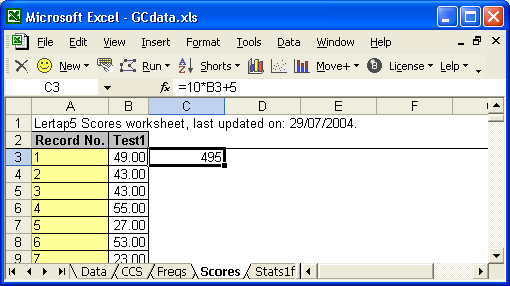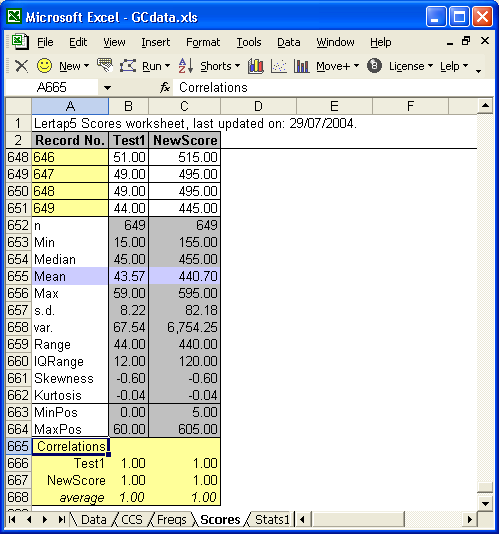﻿ Apply a formula

# Apply a special Scores worksheet formula.

Lertappers sometimes want to create a new score by transforming or combining one or more of the scores found in the Scores worksheet.

For example, let's suppose that a user wanted to apply a linear transformation to one of Scores' scores, of a type commonly found in texts and reference books:

y = mx + b

Here, "x" is a score which already exists, while "m" and "b" are constants.  The new score is "y".

Let's suppose that m=10, and b=5, making the equation

y = 10x + 5

Have a squiz now of the workbook below (taken when running an earlier version of Excel).  The original score, "x" in the equation above, is called Test1, found in Scores column 2.  Get out your best glasses, and look carefully at the Excel Formula Bar, the one which begins with R3C3, and contains a formula which a user entered: =10*RC[-1]+5.

The RC[-1] is Excel's way of referring to a value found in the same row (R), one column to the left (C[-1]).Not everyone likes to work with the R1C1 Excel referencing style seen above in the Formula Bar.  Many users click on Lertap's Excel shortcuts to change the referencing style so that columns are labeled with letters, as shown below:Notice how the formula has changed to =10*B3+5?  B3 refers to the cell where "x", the original score, is found.

Both of these formulas (formulae) say the same thing -- they just use different referencing styles (you can pop back and forth between the referencing styles as much as you wish).

Okay?  We've got a user who wants to make a new score by multiplying the original score, Test1, by 10, and adding 5 to the result.

S/he begins by selecting the cell immediately to the right of the first Test1 score, and then enters the formula by actually typing it in, starting with the equals (=) sign.

After typing the formula, the user presses the <Enter> key, and Excels displays the value of the new score, which in this example is 495.

If this is what's wanted, the user then selects the cell with the new formula by clicking on it, cruises up to the Move+ menu, and clicks on "Apply a special Scores worksheet formula".  Your beloved little Lertap then applies the formula to all other original scores, determines the descriptive statistics related to the new score, and updates the correlation matrix found at the bottom of the Scores worksheet, lo:The label given to the new score, NewScore, may of course be changed.

Don't like the results?  Select the new score's column, delete it, and start again.

Want to know more about working with formulas in Excel?  Look for assistance in Excel Help (there's lots -- you might start by searching Excel Help for "create a formula").